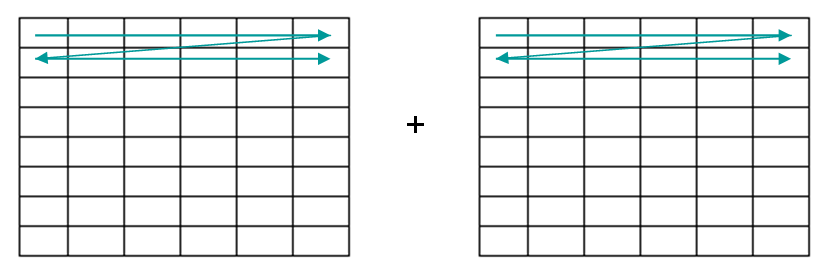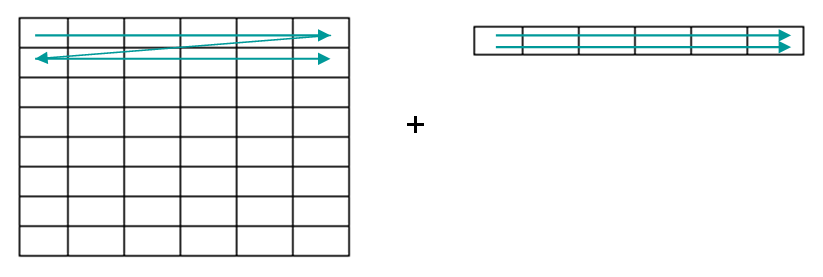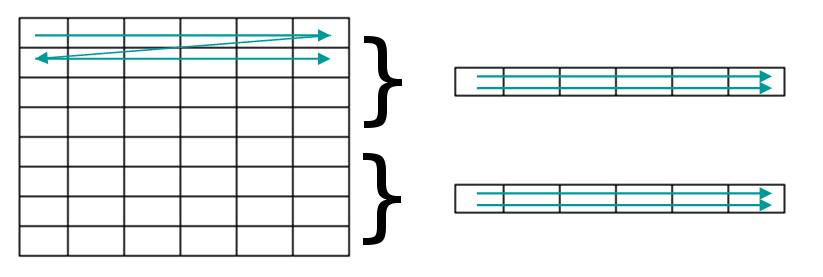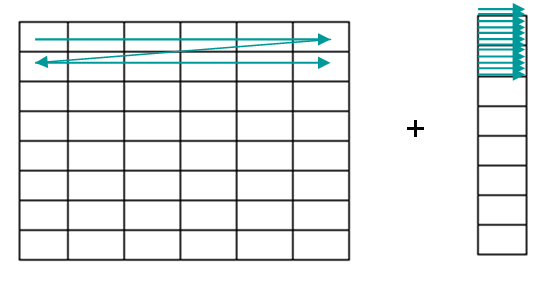$$\renewcommand\AA{\unicode{x212B}}$$

# Divide v1¶

## Summary¶

The Divide algorithm will divide the data values and calculate the corresponding error values of two compatible workspaces.

## Properties¶

Name Direction Type Default Description
LHSWorkspace Input MatrixWorkspace Mandatory The name of the input workspace on the left hand side of the operation
RHSWorkspace Input MatrixWorkspace Mandatory The name of the input workspace on the right hand side of the operation
OutputWorkspace Output MatrixWorkspace Mandatory The name to call the output workspace
AllowDifferentNumberSpectra Input boolean False Are workspaces with different number of spectra allowed? For example, the LHSWorkspace might have one spectrum per detector, but the RHSWorkspace could have its spectra averaged per bank. If true, then matching between the LHS and RHS spectra is performed (all detectors in a LHS spectrum have to be in the corresponding RHS) in order to apply the RHS spectrum to the LHS.
ClearRHSWorkspace Input boolean False For EventWorkspaces only. This will clear out event lists from the RHS workspace as the binary operation is applied. This can prevent excessive memory use, e.g. when subtracting an EventWorkspace from another: memory use will be approximately constant instead of increasing by 50%. At completion, the RHS workspace will be empty.
WarnOnZeroDivide Input boolean True Algorithm usually warns if division by 0 occurs. Set this value to false if one does not want this message appearing

## Description¶

OutputWorkspace = LHSWorkspace / RHSWorkspace


The algorithm will perform the / operation on the data and associated errors from any two compatible workspaces. Workspaces are compatible if:

• The sizes of the two workspaces are compatible (see below)
• If the two workspaces contain Y bins then the values in these must be identical
• the units of the axes match
• the distribution status/counts units match

For information about how errors are handled and propagated see Error Propagation.

### Compatible Sizes¶

 Workspaces are compatible if they are identically sized in which case the values of each cell within each histogram are divided individually. The green arrows shows the order in which the data is divided.They are also compatible if they match the size dimension horizontally, in which case the same values are divided by each histogram in the workspace.If AllowDifferentNumberSpectra is True, then the LHS and RHS spectra are matched using detector IDs. The corresponding spectra are divided by the matching counterpart.If they match in size vertically then the data values will be divided by each bin of the histogram.Finally a workspace containing a single value is compatible to any workspace, in which case the same value will be divided uniformly by every bin in each histogram.

### Operations on EventWorkspaces vs Workspace2Ds¶

LHS / RHS = Output Notes
Workspace2D / Workspace2D = Workspace2D The number of bins DO need to match for both operands.
Workspace2D / EventWorkspace = Workspace2D The histogram representation of the RHS EventWorkspace is used as the right-hand operand. The number of bins DO need to match for both operands.
EventWorkspace / EventWorkspace = EventWorkspace The histogram representation of the RHS EventWorkspace is used as the right-hand operand. The number of bins DO NOT need to match for both operands. The output EventWorkspace will use WeightedEvents, where each event’s weight will have been divided by the Y value at the corresponding X bin on the RHS.
EventWorkspace / Workspace2D = EventWorkspace The histogram representation of the RHS EventWorkspace is used as the right-hand operand. The number of bins DO NOT need to match for both operands.
EventWorkspace / WorkspaceSingleValue = EventWorkspace The value (and error) is applied to the weight of every event in the workspace. The output EventWorkspace will use WeightedEvents. If Output–LHS, then the operation is performed in-place and no copying of workspaces is done.

## Usage¶

Example - Divide as an Algorithm

# create histogram workspaces
dataX1 = [0,1,2,3,4,5,6,7,8,9] # or use dataX1=range(0,10)
dataY1 = [0,1,2,3,4,5,6,7,8] # or use dataY1=range(0,9)
dataE1 = [0,1,2,3,4,5,6,7,8] # or use dataE1=range(0,9)
dataX2 = [0,1,2,3,4,5,6,7,8,9] #X-values must be identical
dataY2 = [2,2,2,2,2,2,2,2,2]
dataE2 = [3,3,3,3,3,3,3,3,3]
ws1 = CreateWorkspace(dataX1, dataY1, dataE1)
ws2 = CreateWorkspace(dataX2, dataY2, dataE2)

# perform the algorithm
ws = Divide(ws1, ws2)

print("The X values are: " + str(ws.readX(0)))
print("The Y values are: " + str(ws.readY(0)))
print("The updated Error values are: " + str(ws.readE(0)))


Output:

The X values are: [ 0.  1.  2.  3.  4.  5.  6.  7.  8.  9.]
The Y values are: [ 0.   0.5  1.   1.5  2.   2.5  3.   3.5  4. ]
The updated Error values are: [ 0.          0.90138782  1.80277564  2.70416346  3.60555128  4.50693909
5.40832691  6.30971473  7.21110255]


Example - Divide as an operator

# create histogram workspaces
dataX1 = [0,1,2,3,4,5,6,7,8,9] # or use dataX1=range(0,10)
dataY1 = [0,1,2,3,4,5,6,7,8] # or use dataY1=range(0,9)
dataE1 = [0,1,2,3,4,5,6,7,8] # or use dataE1=range(0,9)
dataX2 = [0,1,2,3,4,5,6,7,8,9] #X-values must be identical
dataY2 = [2,2,2,2,2,2,2,2,2]
dataE2 = [3,3,3,3,3,3,3,3,3]
ws1 = CreateWorkspace(dataX1, dataY1, dataE1)
ws2 = CreateWorkspace(dataX2, dataY2, dataE2)

# perform the algorithm
ws = ws1 / ws2

print("The X values are: " + str(ws.readX(0)))
print("The Y values are: " + str(ws.readY(0)))
print("The updated Error values are: " + str(ws.readE(0)))


Output:

The X values are: [ 0.  1.  2.  3.  4.  5.  6.  7.  8.  9.]
The Y values are: [ 0.   0.5  1.   1.5  2.   2.5  3.   3.5  4. ]
The updated Error values are: [ 0.          0.90138782  1.80277564  2.70416346  3.60555128  4.50693909
5.40832691  6.30971473  7.21110255]


Example - Divide using in-place operator

# create histogram workspaces
dataX1 = [0,1,2,3,4,5,6,7,8,9] # or use dataX1=range(0,10)
dataY1 = [0,1,2,3,4,5,6,7,8] # or use dataY1=range(0,9)
dataE1 = [0,1,2,3,4,5,6,7,8] # or use dataE1=range(0,9)
dataX2 = [0,1,2,3,4,5,6,7,8,9] #X-values must be identical
dataY2 = [2,2,2,2,2,2,2,2,2]
dataE2 = [3,3,3,3,3,3,3,3,3]
ws = CreateWorkspace(dataX1, dataY1, dataE1)
ws1 = CreateWorkspace(dataX2, dataY2, dataE2)

# perform the algorithm
ws /= ws1

print("The X values are: " + str(ws.readX(0)))
print("The Y values are: " + str(ws.readY(0)))
print("The updated Error values are: " + str(ws.readE(0)))


Output:

The X values are: [ 0.  1.  2.  3.  4.  5.  6.  7.  8.  9.]
The Y values are: [ 0.   0.5  1.   1.5  2.   2.5  3.   3.5  4. ]
The updated Error values are: [ 0.          0.90138782  1.80277564  2.70416346  3.60555128  4.50693909
5.40832691  6.30971473  7.21110255]


Example - Divide by a scalar

# create histogram workspaces
dataX1 = [0,1,2,3,4,5,6,7,8,9] # or use dataX1=range(0,10)
dataY1 = [0,1,2,3,4,5,6,7,8] # or use dataY1=range(0,9)
dataE1 = [0,1,2,3,4,5,6,7,8] # or use dataE1=range(0,9)
ws1 = CreateWorkspace(dataX1, dataY1, dataE1)

# perform the algorithm
ws = ws1 / 2.5

print("The X values are: " + str(ws.readX(0)))
print("The Y values are: " + str(ws.readY(0)))
print("The updated Error values are: " + str(ws.readE(0)))


Output:

The X values are: [ 0.  1.  2.  3.  4.  5.  6.  7.  8.  9.]
The Y values are: [ 0.   0.4  0.8  1.2  1.6  2.   2.4  2.8  3.2]
The updated Error values are: [ 0.   0.4  0.8  1.2  1.6  2.   2.4  2.8  3.2]


Categories: AlgorithmIndex | Arithmetic